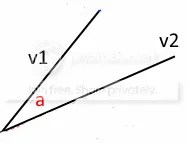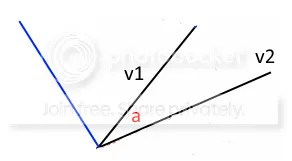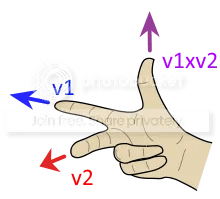Read my previous post on dot products first!

A dot product v1 . v2 tells you how much of v1 is going in the same direction as v2 (or vice versa).

Cross products (written as v1 x v2) are about different directions. They tell you two things

• how much of v1 is going in a direction 90 degrees (ie away) from v2, and
• the direction which is at 90 degrees to both v1 and v2

I’m going to need to explain this in more detail.#### 1. How much of v1 is going in a direction 90 degrees from v2?

The dot product v1 . v2 = |v1||v2| cos(a) is the lengths of v1 and v2, multiplied by each other, and by cos of the angle between them. So the closer the vectors are together, the smaller the angle, and the bigger the dot product.

Suppose instead that we wanted to measure how different v1 and v2 were. We could use the same formula, but with sin instead of cos – because this will give us a small value when the angle is small (the vectors are close), and a large value when the angle is large (the vectors are going in quite different directions).

So let’s calculate this difference as  |v1||v2| sin(a)

And this is the first part of the formula for the cross product.

#### 2. What is the direction which is at 90 degrees to both v1 and v2?

There is only one direction which is at right angles (90 degrees) to both v1 and v2. Together with v1 and v2, it makes up a 3D space.

If you think of the picture above as showing a flat surface (or plane, in math language), then the direction we are looking for is shown by the blue line below.So for example, if you think of v1 as being the x axis, and v2 as being the y axis, then the blue vector is the z axis.

As another example, if you think of the Codea screen, with 0,0 in the bottom left corner, x running from left to right, and y running from bottom to top, then the third dimension, z, runs straight into the screen (and out towards us) – which is what you get if you turn on 3D with the perspective command.

This third direction I keep talking about needs a name – and it has one – the normal vector.

#### The “normal” vector

If you have a flat surface (plane) formed by two vectors, as above, the normal vector is the direction which is at right angles to both the other vectors. So when I talk about “the normal”, I mean the direction that is perpendicular to a plane (two other vectors), ie the blue line above.

If you are in 3D, the normal to a surface formed by any two of the dimensions (eg x and y) is simply the third dimension.

Why is it called normal? That is just weird. The answer is that it comes from the Latin normalis, meaning right-angled (“made according to the square”). That’s fine, but it’s pretty misleading, because “normal” doesn’t mean anything like that now.

Normal is not normalised

Normal vectors are usually normalised, so they have a length of 1. Unfortunately, “normalised” means something completely different from “normal”.

I explained it in my earlier post on vectors, but essentially, all vectors consist of length and direction, and you can separate them, by

• calculating their length,
• dividing the vector by that length, to get direction
• the direction has a length of 1 (obviously)
• and this is called a unit vector, or “normalised”

Why the word normalised? It means to “make normal”, or perhaps, “to standardise”, which gives us a clue to why it is used. We need to “standardise” direction vectors to have a length of 1.

It’s just a pity that we have to use both words together, eg normalised normal vector! Just remember they are completely different.

### 3. The cross product

The cross product is a 3D vector with

• size (my “difference” formula above), and
• direction (the normal vector of v1 and v2) and

So v1 x v2 =  |v1||v2| sin(a) * normal vector

How do we get the normal vector? Well, there is no need, because there is a formula which uses the x,y,z values of the vectors

```v1 = (x1, y1, z1)
v2 = (x2, y2, z2)
CrossProduct = (y1*z2-y2*z1, z1*x2-z2*x1, x1*y2, x2*y1)
--but Codea makes it easy with a function
CrossProduct = v1:cross(v2)
```

and we can use this to get the cross product. Then we can do all sorts of things with it

• find the normal to a plane (very useful for lighting mesh triangles in 3D)
• how much of v1 is pointing away from v2
• electrical charges
• mechanics

One last thing. The normal vector could point up, or point down. Which way is it?

OpenGL is what is called right handed, which is because you can use your right hand to help remember which direction is used. For cross products, if you hold your hand as shown below, with one finger pointing along v1, and another along v2, then your thumb will point along the normal.From → 3D, Graphics, Programming